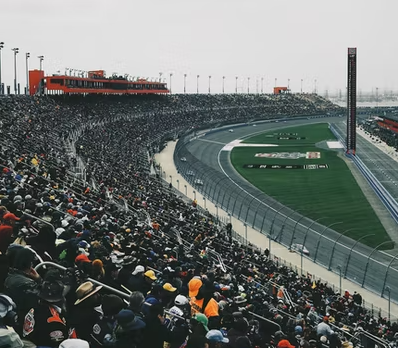## Banked Curve

Track curves on race courses are steeply banked, which allow race car drivers to taken them at very fast speeds. If a particular course has a 125 m radius curve banked at 50°, what is the max allowable speed if the road is frictionless?Hint
$$tan\theta =\frac{v^2}{rg}$$$where $$\theta$$ is the banked angle, $$v$$ is the velocity, $$g$$ is the acceleration due to gravity, and $$r$$ is the radius. Hint 2 Use the equation to solve for velocity, $$v$$ . Start with: $$tan\theta =\frac{v^2}{rg}$$$
where $$\theta$$ is the banked angle, $$v$$ is the velocity, $$g$$ is the acceleration due to gravity, and $$r$$ is the radius. Thus,
$$tan50^{\circ} =\frac{v^2}{(125m)(9.8m/s^2)}$$$Solving for velocity, $$v=\sqrt{(125m)(9.8m/s^2)\times tan50^{\circ}}=\sqrt{(1.19)(1,225)m^2/s^2}$$$
$$v=\sqrt{1,457.75m^2/s^2}=38.2\:m/s$$\$
38.2 m/s